Test: Digital Filters Design Consideration

# Test: Digital Filters Design Consideration

Test Description

## 10 Questions MCQ Test Signals and Systems | Test: Digital Filters Design Consideration

Test: Digital Filters Design Consideration for Electrical Engineering (EE) 2023 is part of Signals and Systems preparation. The Test: Digital Filters Design Consideration questions and answers have been prepared according to the Electrical Engineering (EE) exam syllabus.The Test: Digital Filters Design Consideration MCQs are made for Electrical Engineering (EE) 2023 Exam. Find important definitions, questions, notes, meanings, examples, exercises, MCQs and online tests for Test: Digital Filters Design Consideration below.
Solutions of Test: Digital Filters Design Consideration questions in English are available as part of our Signals and Systems for Electrical Engineering (EE) & Test: Digital Filters Design Consideration solutions in Hindi for Signals and Systems course. Download more important topics, notes, lectures and mock test series for Electrical Engineering (EE) Exam by signing up for free. Attempt Test: Digital Filters Design Consideration | 10 questions in 10 minutes | Mock test for Electrical Engineering (EE) preparation | Free important questions MCQ to study Signals and Systems for Electrical Engineering (EE) Exam | Download free PDF with solutions
 1 Crore+ students have signed up on EduRev. Have you?
Test: Digital Filters Design Consideration - Question 1

### The ideal low pass filter cannot be realized in practice.

Detailed Solution for Test: Digital Filters Design Consideration - Question 1

Explanation: We know that the ideal low pass filter is non-causal. Hence, a ideal low pass filter cannot be realized in practice.

Test: Digital Filters Design Consideration - Question 2

### The following diagram represents the unit sample response of which of the following filters?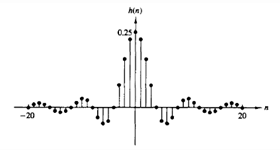Detailed Solution for Test: Digital Filters Design Consideration - Question 2

Explanation: At n=0, the equation for ideal low pass filter is given as h(n)=ω/π.
From the given figure, h(0)=0.25=>ω=π/4.
Thus the given figure represents the unit sample response of an ideal low pass filter at ω=π/4.

Test: Digital Filters Design Consideration - Question 3

### If h(n) has finite energy and h(n)=0 for n<0, then which of the following are true?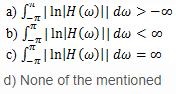Detailed Solution for Test: Digital Filters Design Consideration - Question 3

Explanation: If h(n) has finite energy and h(n)=0 for n<0, then according to the Paley-Wiener theorem, we have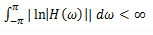Test: Digital Filters Design Consideration - Question 4

If |H(ω)| is square integrable and if the integral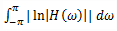is finite, then the filter with the frequency response

Detailed Solution for Test: Digital Filters Design Consideration - Question 4

Explanation: If |H(ω)| is square integrable and if the integral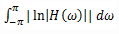is finite, then we can associate with |H(ω)| and a phase response θ(ω), so that the resulting filter with the frequency response H(ω)=|H(ω)|ejθ(ω) is causal.

Test: Digital Filters Design Consideration - Question 5

The magnitude function |H(ω)| can be zero at some frequencies, but it cannot be zero over any finite band of frequencies.

Detailed Solution for Test: Digital Filters Design Consideration - Question 5

Explanation: One important conclusion that we made from the Paley-Wiener theorem is that the magnitude function |H(ω)| can be zero at some frequencies, but it cannot be zero over any finite band of frequencies, since the integral then becomes infinite. Consequently, any ideal filter is non-causal.

Test: Digital Filters Design Consideration - Question 6

If h(n) is causal and h(n)=he(n)+ho(n),then what is the expression for h(n) in terms of only he(n)?

Detailed Solution for Test: Digital Filters Design Consideration - Question 6

Explanation: Given h(n) is causal and h(n)= he(n)+ho(n)
=>he(n)=1/2[h(n)+h(-n)] Now, if h(n) is causal, it is possible to recover h(n) from its even part he(n) for 0≤n≤∞ or from its odd component ho(n) for 1≤n≤∞.
=>h(n)= 2he(n)u(n)-he(0)δ(n) ,n ≥ 0.

Test: Digital Filters Design Consideration - Question 7

If h(n) is causal and h(n)=he(n)+ho(n),then what is the expression for h(n) in terms of only ho(n)?

Detailed Solution for Test: Digital Filters Design Consideration - Question 7

Explanation: Given h(n) is causal and h(n)= he(n)+ho(n)
=>he(n)=1/2[h(n)+h(-n)] Now, if h(n) is causal, it is possible to recover h(n) from its even part he(n) for 0≤n≤∞ or from its odd component ho(n) for 1≤n≤∞.
=>h(n)= 2ho(n)u(n)+h(0)δ(n) ,n ≥ 1
since ho(n)=0 for n=0, we cannot recover h(0) from ho(n) and hence we must also know h(0).

Test: Digital Filters Design Consideration - Question 8

If h(n) is absolutely summable, i.e., BIBO stable, then the equation for the frequency response H(ω) is given as?

Detailed Solution for Test: Digital Filters Design Consideration - Question 8

Explanation: . If h(n) is absolutely summable, i.e., BIBO stable, then the frequency response H(ω) exists and
H(ω)= HR(ω)+j HI(ω)
where HR(ω) and HI(ω) are the Fourier transforms of he(n) and ho(n) respectively.

Test: Digital Filters Design Consideration - Question 9

HR(ω) and HI(ω) are interdependent and cannot be specified independently when the system is causal.

Detailed Solution for Test: Digital Filters Design Consideration - Question 9

Explanation: Since h(n) is completely specified by he(n), it follows that H(ω) is completely determined if we know HR(ω). Alternatively, H(ω) is completely determined from HI(ω) and h(0). In short, HR(ω) and HI(ω) are interdependent and cannot be specified independently when the system is causal.

Test: Digital Filters Design Consideration - Question 10

The frequency ωP is called as:

Detailed Solution for Test: Digital Filters Design Consideration - Question 10

Explanation: Pass band edge ripple is the frequency at which the pass band starts transiting to the stop band.

## Signals and Systems

32 videos|76 docs|63 tests
 Use Code STAYHOME200 and get INR 200 additional OFF Use Coupon Code
Information about Test: Digital Filters Design Consideration Page
In this test you can find the Exam questions for Test: Digital Filters Design Consideration solved & explained in the simplest way possible. Besides giving Questions and answers for Test: Digital Filters Design Consideration, EduRev gives you an ample number of Online tests for practice

## Signals and Systems

32 videos|76 docs|63 tests